# Modern Physics Modern physics means physics based on

• Slides: 12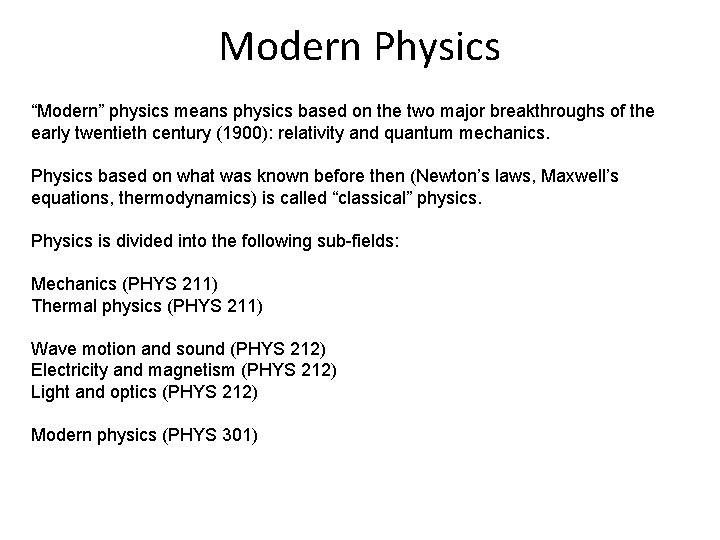Modern Physics “Modern” physics means physics based on the two major breakthroughs of the early twentieth century (1900): relativity and quantum mechanics. Physics based on what was known before then (Newton’s laws, Maxwell’s equations, thermodynamics) is called “classical” physics. Physics is divided into the following sub-fields: Mechanics (PHYS 211) Thermal physics (PHYS 211) Wave motion and sound (PHYS 212) Electricity and magnetism (PHYS 212) Light and optics (PHYS 212) Modern physics (PHYS 301)Newtonian Mechanics • The relation between a force and the acceleration it causes was first understood by Isaac Newton (1642– 1727). • The study of that relation, as Newton presented it, is called Newtonian mechanics. • Newtonian mechanics does not apply to all situations. If the speeds of the interacting bodies are very large—an appreciable fraction of the speed of light— we must replace Newtonian mechanics with Einstein’s theory of relativity, which holds at any speed, including those near the speed of light. • If the interacting bodies are on the scale of atomic structure (for example, they might be electrons in an atom), we must replace Newtonian mechanics with quantum mechanics. • Physicists now view Newtonian mechanics as a special case of these two more comprehensive theories.Fundamental Constants • http: //hyperphysics. phyastr. gsu. edu/hbase/tables/funcon. html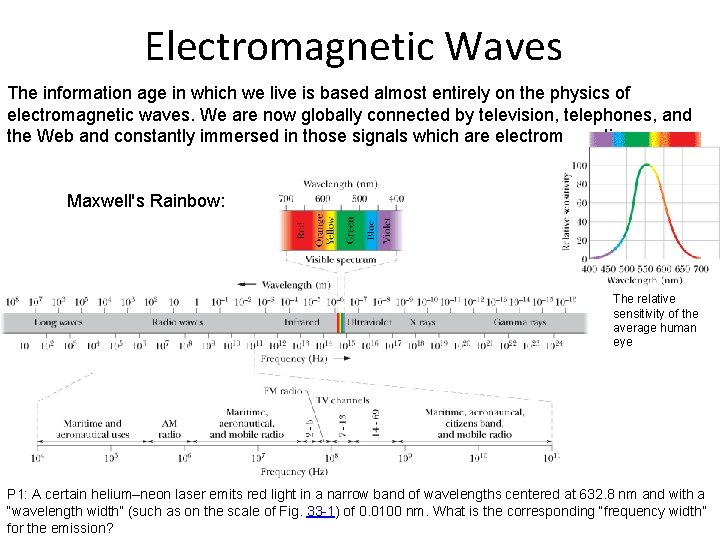Electromagnetic Waves The information age in which we live is based almost entirely on the physics of electromagnetic waves. We are now globally connected by television, telephones, and the Web and constantly immersed in those signals which are electromagnetic waves. Maxwell's Rainbow: The relative sensitivity of the average human eye P 1: A certain helium–neon laser emits red light in a narrow band of wavelengths centered at 632. 8 nm and with a “wavelength width” (such as on the scale of Fig. 33 -1) of 0. 0100 nm. What is the corresponding “frequency width” for the emission?Electromagnetic Wave 1. 2. 3. 4. The electric and magnetic fields are always perpendicular to the direction in which the wave is traveling. Thus, the wave is a transverse wave. The electric field is always perpendicular to the magnetic field. The cross product, always gives the direction in which the wave travels. The fields always vary sinusoidally, just like the transverse waves, the fields vary with the same frequency and in phase (in step) with each other.Boat Race in a River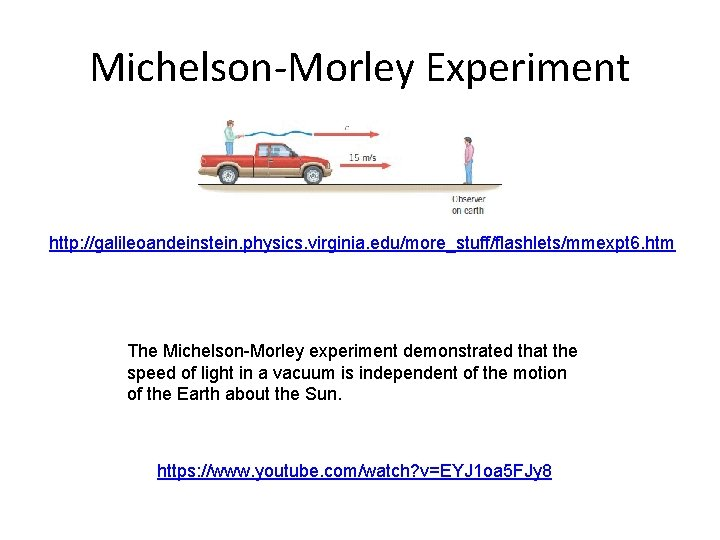Michelson-Morley Experiment http: //galileoandeinstein. physics. virginia. edu/more_stuff/flashlets/mmexpt 6. htm The Michelson-Morley experiment demonstrated that the speed of light in a vacuum is independent of the motion of the Earth about the Sun. https: //www. youtube. com/watch? v=EYJ 1 oa 5 FJy 8Einstein • Many people think that Albert Einstein (1879– 1955) was the greatest physicist of the 20 th century. Not only did he develop modern relativity, thus revolutionizing our concept of the universe, he also made fundamental contributions to the foundations of quantum mechanics. (credit: The Library of Congress)Relativity is the study of how different observers measure the same event. The word relativity might conjure an image of Einstein, but the idea did not begin with him. People have been exploring relativity for many centuries. Galileo and Newton developed the first correct version of classical relativity. Einstein developed the modern theory of relativity. Modern relativity is divided into two parts. Special relativity deals with observers who are moving at constant velocity. General relativity deals with observers who are undergoing acceleration. Einstein is famous because his theories of relativity made revolutionary predictions. Most importantly, his theories have been verified to great precision in a vast range of experiments, altering forever our concept of space and time.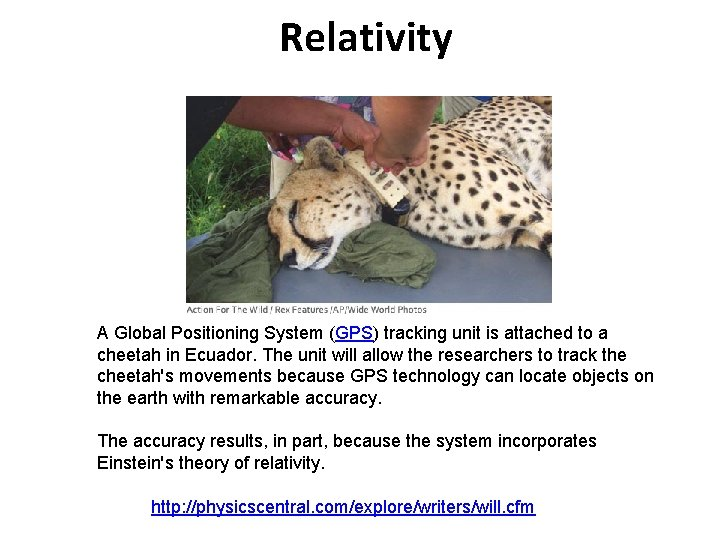Relativity A Global Positioning System (GPS) tracking unit is attached to a cheetah in Ecuador. The unit will allow the researchers to track the cheetah's movements because GPS technology can locate objects on the earth with remarkable accuracy. The accuracy results, in part, because the system incorporates Einstein's theory of relativity. http: //physicscentral. com/explore/writers/will. cfm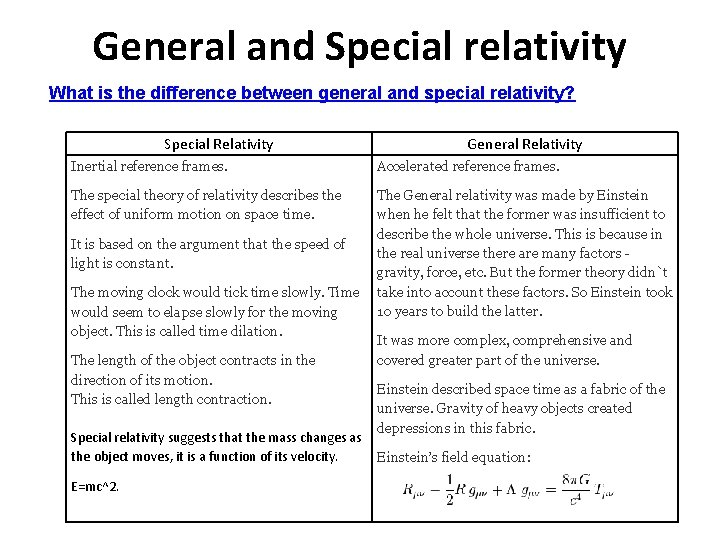General and Special relativity What is the difference between general and special relativity? Special Relativity General Relativity Inertial reference frames. Accelerated reference frames. The special theory of relativity describes the effect of uniform motion on space time. The General relativity was made by Einstein when he felt that the former was insufficient to describe the whole universe. This is because in the real universe there are many factors - gravity, force, etc. But the former theory didn`t take into account these factors. So Einstein took 10 years to build the latter. It is based on the argument that the speed of light is constant. The moving clock would tick time slowly. Time would seem to elapse slowly for the moving object. This is called time dilation. The length of the object contracts in the direction of its motion. This is called length contraction. It was more complex, comprehensive and covered greater part of the universe. Einstein described space time as a fabric of the universe. Gravity of heavy objects created depressions in this fabric. Special relativity suggests that the mass changes as the object moves, it is a function of its velocity. Einstein’s field equation: E=mc^2.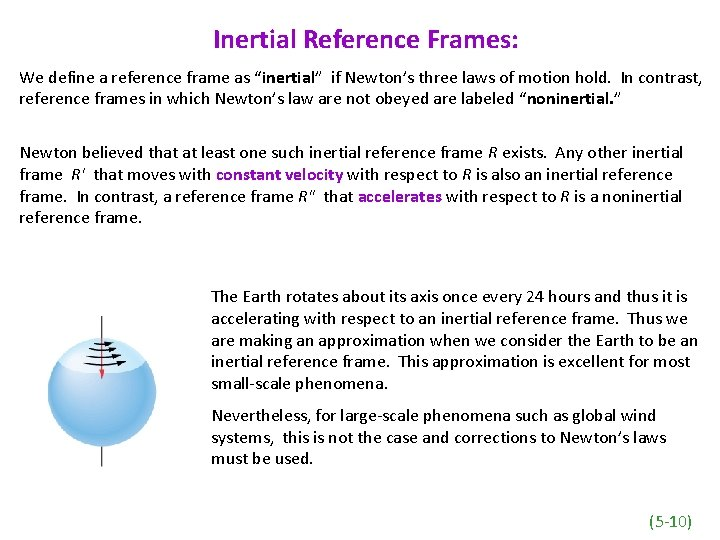Inertial Reference Frames: We define a reference frame as “inertial” if Newton’s three laws of motion hold. In contrast, reference frames in which Newton’s law are not obeyed are labeled “noninertial. ” Newton believed that at least one such inertial reference frame R exists. Any other inertial frame R' that moves with constant velocity with respect to R is also an inertial reference frame. In contrast, a reference frame R" that accelerates with respect to R is a noninertial reference frame. The Earth rotates about its axis once every 24 hours and thus it is accelerating with respect to an inertial reference frame. Thus we are making an approximation when we consider the Earth to be an inertial reference frame. This approximation is excellent for most small-scale phenomena. Nevertheless, for large-scale phenomena such as global wind systems, this is not the case and corrections to Newton’s laws must be used. (5 -10)